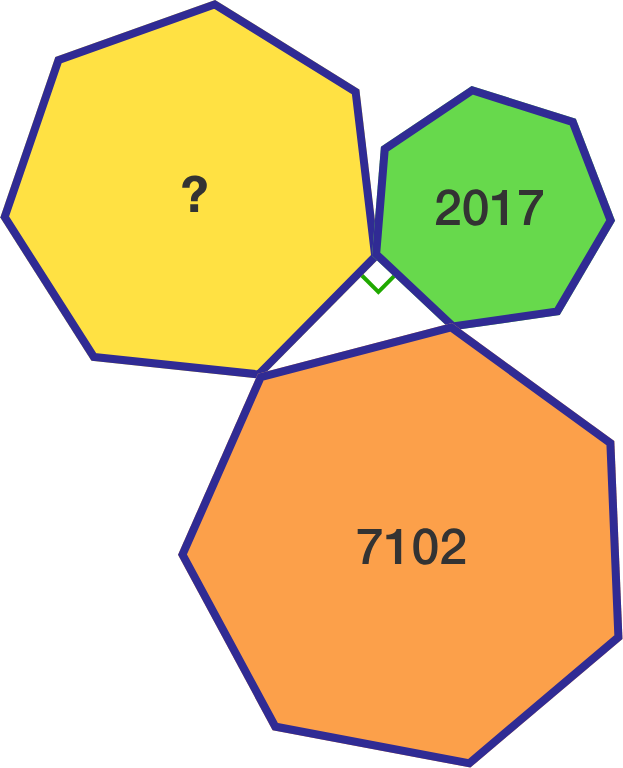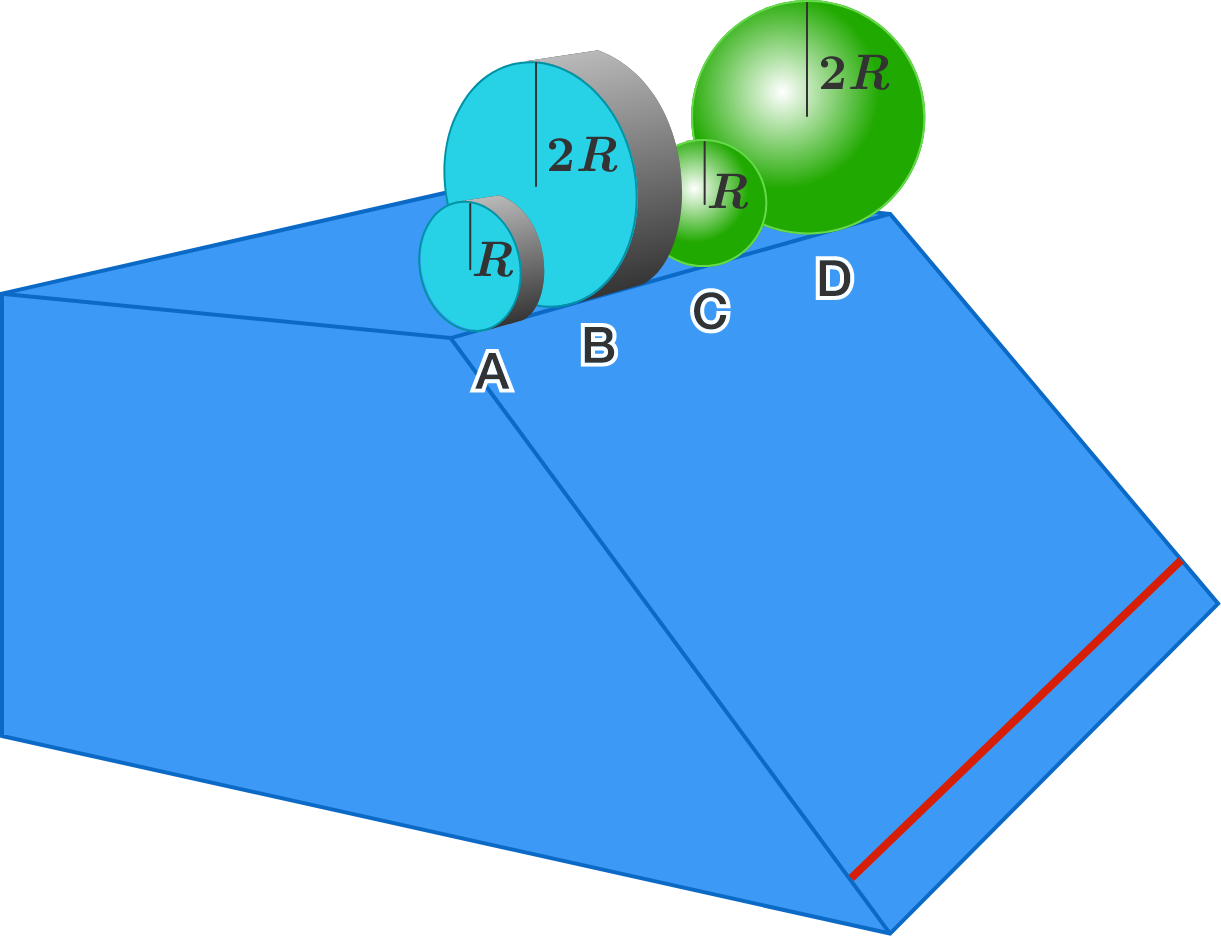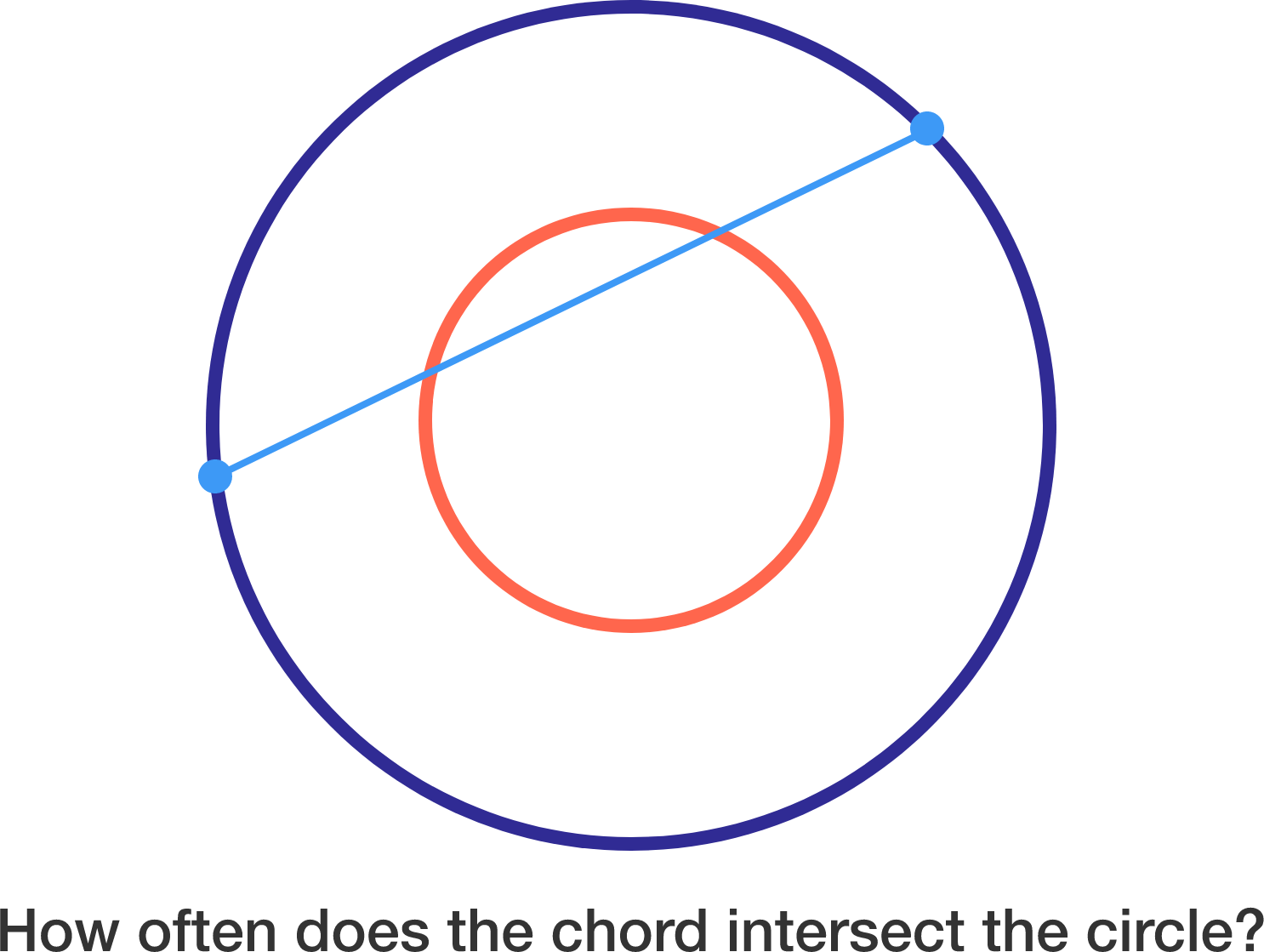# Problems of the Week

Contribute a problem

# 2017-05-01 Intermediate

$\begin{array} { l l l } & A & B \\ & C & D \\ + & E & F \\ \hline & G & H \\ \end{array}$

In the above cryptogram, all the letters represent distinct digits from 1 to 9.

What is the minimum possible value of $\overline{GH}$?

For a greater challenge, try Cryptotastic #2.

$\begin{array} { l l l l l l l l } & A & B & C & D & E & 9 \\ \times & & & & & & 4 \\ \hline & 9 & A & B & C & D & E \\ \end{array}$

I have a 6-digit positive integer with a last digit 9. If I move its last digit directly to the front without shifting the other digits, then this new 6-digit number is 4 times the original one.

What is the original 6-digit number?Three regular heptagons with different side lengths form a right triangle, using one side of each. The smallest heptagon has area $2017 \text{ cm}^2,$ while the largest one has area $7102 \text{ cm}^2.$

Find the area of the remaining heptagon in $\text{cm}^2.$4 objects $A, B, C, D$ are made up of the same material. They are released, simultaneously from rest, from the starting line on the top of a rough inclined plane, so that they roll without slipping. $A$ and $B$ are cylinders, and $C$ and $D$ are spheres.

What is the order relation of the times taken by the objects to cross the finish line?
(A, B, C, D in the answer options denote the times taken by corresponding objects.)


Details and Assumptions:

• The objects are released with all their centers above the same starting line, which is the top edge of the ramp. They are considered to have crossed the finish line--the red line near the bottom edge--only if their centers have crossed the line when viewed from the side.

• Moment of inertia of a cylinder is $\frac{1}{2} MR^2$ and that of a sphere is $\frac{2}{5} MR^2$, where $M$ is the mass and $R$ is the radius of the respective objects.

• $B$ has twice the radius of $A,$ and $D$ has twice the radius of $C$.

Consider concentric circles with respective radii $\pi$ and $2\pi$. Choose two points on the larger circle independently and uniformly at random, and then join them to form a chord. If $P$ is the probability that this chord intersects the smaller circle at at least one point, then find $\lfloor 1000P \rfloor$.Notation: $\lfloor \cdot \rfloor$ denotes the floor function.

×

Problem Loading...

Note Loading...

Set Loading...